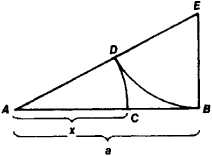# Golden Section

Also found in: Dictionary, Thesaurus, Idioms, Wikipedia.

## Golden Section,

in mathematics, division of a line segment into two segments such that the ratio of the original segment to the larger division is equal to the ratio of the larger division to the smaller division. If c is the original segment, b is the larger division, and a is the smaller division, then c = a + b and c/b = b/a. Thus, b is the geometric mean of a and c; the ratio is known as the Divine Proportion. The Golden Rectangle, whose length and width are the segments of a line divided according to the Golden Section, occupies an important position in painting, sculpture, and architecture, because its proportions have long been considered the most attractive to the eye. The constructions of regular polygons of 5, 10, and 15 sides depend on the division of a line by the Golden Section. The numerical ratio of the greater segment of the line to the shorter segment as determined by the Golden Section is symbolized by the Greek letter phi and has the approximate value 1.618. It occurs in many widely varying areas of mathematics. For example, in the Fibonacci sequence (the sequence of numbers formed by adding successive members to find the next member—0, 1, 1, 2, 3, 5, 8, 13, … ), the values of the ratios 1, 2/1, 3/2, 5/3, 8/5, 13/8, … approach the value of the Golden Section.

### Bibliography

See H. E. Huntley, The Divine Proportion (1970).

## Golden Section

the division of a line segment AB into two parts in such a way that its larger part AC is the mean proportional between the entire segment AB and its smaller part CB (see Figure 1).Figure 1

The algebraic determination of the golden section of the segment AB = a reduces to solving the equation a/x = x/(ax) (where x = AC), from which x = a (− 1)/2 ≈ 0.62a. The ratio of x to a may also be expressed approximately by the fractions ⅔, ⅗, ⅝, 8/13, 13/21, and so on, where 2, 3, 5, 8, 13, 21, and so forth are Fibonacci numbers. The geometric construction of the golden section of the segment AB is accomplished in the following manner: a perpendicular to AB is drawn at point B, the segment BE = 1/2AB is measured off, A and E are connected, ED = EB is measured off, and finally, AC = AD, thus AB/AC = AC/CB. The golden section was already known in antiquity. In the classical literature that has come down to us, the golden section is first encountered in Euclid’s Elements (third century B.C.). The term “golden section” was introduced by Leonardo da Vinci (the turn of the 16th century). The principles of the golden section and the proportional relationships close to it have served as the basis for the compositional construction of many works of world art (chiefly architectural works of ancient Greece and Rome and the Renaissance).

## golden section

[′gōl·dən ′sek·shən]
(mathematics)
The division of a line so that the ratio of the whole line to the larger interval equals the ratio of the larger interval to the smaller. Also known as divine proportion; extreme and mean ratio; golden mean; golden ratio.

## golden section

The division of a line into two segments so that the ratio of the whole line to the larger segment equals the ratio of larger segment to the smaller one; often called the golden section. This ratio was once considered by some as having an inherent aesthetic value.
References in periodicals archive ?
Results in Tables 5 and 6 show that all mean values [M.sub.r] of the golden section ratios [h.sub.B]/h for all groups of respondents (for both genders and for all age groups of tram drivers) deviate very little from the theoretical value of the golden section which is 0.618.
The following summary of the golden section method (GSM) is of description for minimizing a strictly quasi-convex function over the uncertain interval ([a.sub.1], [b.sub.1]) .
The golden section method does not require gradient information.
In our opinion Fibonacci Spiral  is the golden section in the two dimensional world.
Besides, amplitude error is considered, and golden section algorithm is directly used to search the error.
However, it is not until the 19th century when the first empirical study about the relevance of golden section on aesthetic perceptions is reported (Fechner, 1876/1997).
We assume that each groove of the Fresnel lens has a different design main wavelength and use the golden section method to search the optimal design main wavelength.
A quasigroup (Q, x) is said to be a golden section quasigroup or shortly a GS-quasigroup  if it satisfies the (mutually equivalent) identities
It is clear, that first irrational maxima must be connected with golden section. The question is about the rest of the maxima.
Zuffi, an art historian and author, presents double-page spreads of important paintings to explain many key concepts in Renaissance art, from perspective to the golden section to fresco to symbolism.
The ancient Greeks used the Golden Section as the perfect proportion for sculpture and architecture.

Site: Follow: Share:
Open / Close